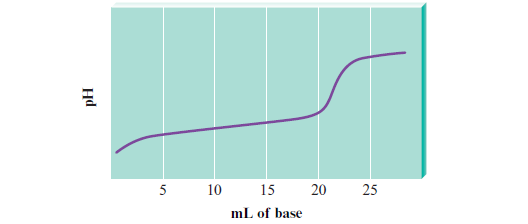# Problem: Consider the titration of a generic weak acid HA with a strong base that gives the following titration curve:On the curve, indicate the points that correspond to the following:f. pH depends only on the amount of excess strong base added

###### FREE Expert Solution

pH depends only on the amount of excess strong base added

• generic weak acid HA is consumed
• only strong base is present
• beyond equivalence point###### Problem Details

Consider the titration of a generic weak acid HA with a strong base that gives the following titration curve:On the curve, indicate the points that correspond to the following:

f. pH depends only on the amount of excess strong base added